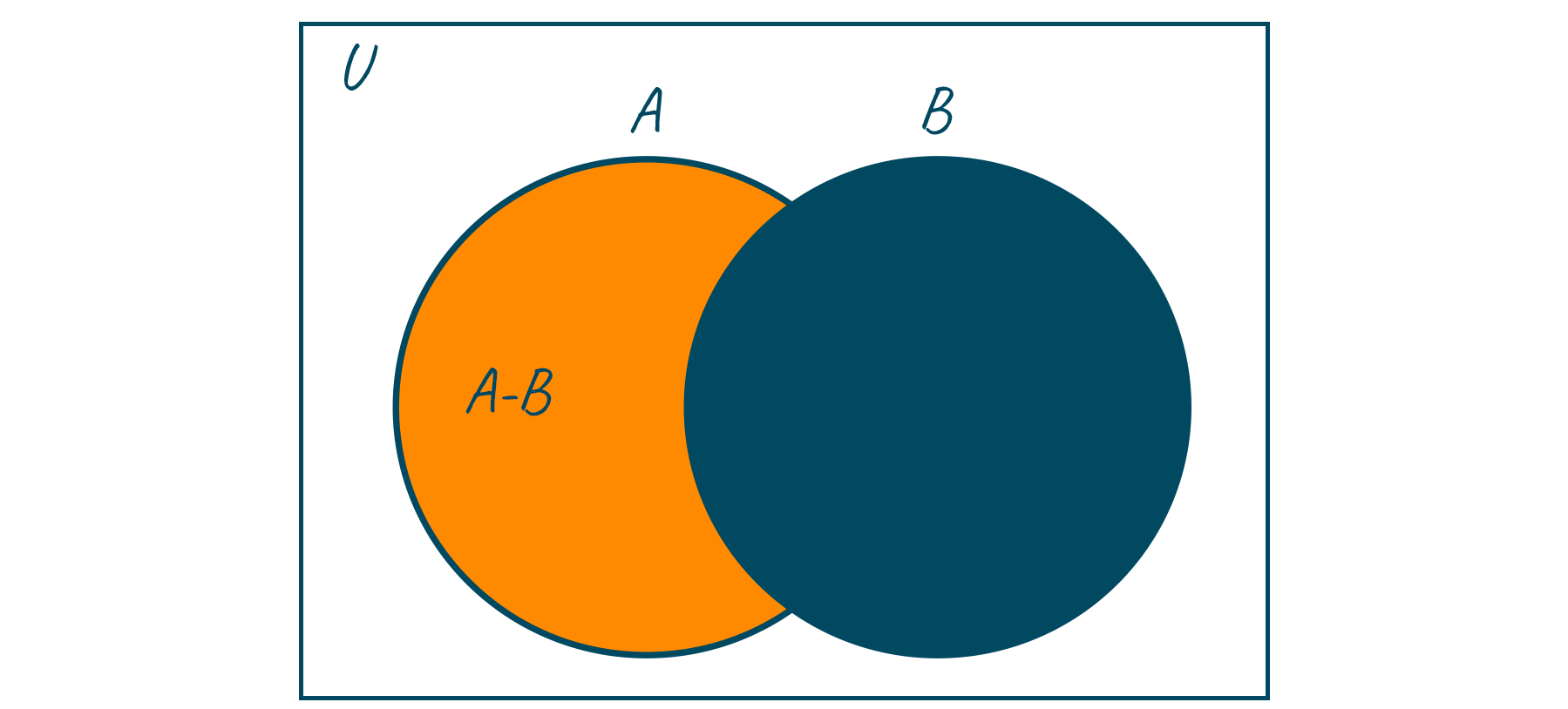# Python Set Difference with Examples and Code

Set theory, a major domain in Mathematics, involves housing a group or collection of related elements. Seeing the importance of mathematical sets, Python decided to make the implementation of the mathematical sets an inbuilt data structure. In this article, we will learn how to find the difference between two set objects. But before that let us have a quick recap of what sets are and what we mean when we say find the difference of sets.

## What is a Set in Python?

Sets are one of the four major data structures in python and are represented using curly braces. Set, like a list, can store all forms of data (Strings, integer, float) and even a combination of different data types. But logically it makes sense to store only related objects together in a set. Now you may be wondering if both dictionary and set data structures use curly braces to represent themselves, then what is the difference between them? Well, the answer is pretty simple, Dictionary stores data in the form of key-value pair, whereas this is not the case in sets.

Let us now understand Sets from a more technical viewpoint. To be precise, sets are mutable, iterable and do not allow duplicate elements inside them. An important point to remember is that since sets are unordered, therefore we cannot access its elements using indexes as we can in lists. Actually, Sets have a major advantage in comparison to other data structures. Sets internally make use of a data structure called the hash table, due to which they contain a highly optimized method for checking whether a specific element is contained in the set or not. This method is even faster than the fastest searching algorithm. Let us now look at a few examples of sets in python

For example:

```setA = { "a", "b", "c"}
print(setA)
# Adding element to the set
print(setA)
```

Output:

```{'c', 'b', 'a'}
{'c', 'd', 'b', 'a'}
```

## What is the Difference Between Two Sets?

When we say find the difference between Set A and Set B, what we need is to find those elements that are present in set A but not in Set B. Mathematically this is represented using the – operator. Have a look below to understand this concept better. The left-hand side is the mathematical notation and the right-hand side is the meaning of the notation.

```A – B: elements present in A, but not in B
B – A: elements present in B, but not in A
```

Let us now take an example to solidify this knowledge

```If A = { 23, 453, 43, 66, 21} and B = { 43, 785, 12, 35, 23, 545}
Then A – B = { 453, 66, 21 }
And B – A = { 785, 12, 35, 545}
```

We can also visualize this concept using a Venn diagram. Look at the image below.## How to Find Difference Between Two Sets in Python?

We can find python set difference using 2 methods. Let us look in detail at both the methods along with some examples.

### 1) Using Set.difference() method

This is an inbuilt function suitable for finding the difference between any two given sets. It implements the difference operator (-) for sets. This method is called upon the set object from which elements are to be subtracted. It takes one argument, that is the set whose elements are to be subtracted from the set on which the function is called.

So, to calculate A-B, the function call will look something like this A.difference(B). Similarly, to calculate B- A, the function call will be B.difference(A). We hope that till now everything is clear.
Now, coming to the value returned by this function. This method returns a new set that contains the resulting elements from the difference between two sets. Note that, this method does not modify the original sets. Let us now look at an example to see this function in action.

For example:

```# Set A
A = { 23, 453, 43, 66, 21}
# Set B
B =  { 43, 785, 12, 35, 23, 545}

C = A.difference(B) # A - B
D = B.difference(A) # B - A

# Display statements
print("A - B : " +str(C))
print("B - A : " +str(D))
```

Output:

```A - B : {66, 21, 453}
B - A : {545, 35, 12, 785}
```

### 2) Using – Operator

This method is much simpler than the previous method and makes use of the subtraction operator.

For example:

```# Set A
A = { 23, 453, 43, 66, 21}
# Set B
B =  { 43, 785, 12, 35, 23, 545}

C = A - B
D = B - A

# Display statements
print("A - B : " +str(C))
print("B - A : " +str(D))
```

Output:

```A - B : {66, 21, 453}
B - A : {545, 35, 12, 785}
```

## Conclusion

Python is known for its easy, English-like syntax. Python provides us with a large bag of in-built functions using which we can perform many major operations of the sets. In this article, we discussed the difference operator of sets and its implementation in python. You can also learn about the intersection operator of sets

### FavTutor - 24x7 Live Coding Help from Expert Tutors!